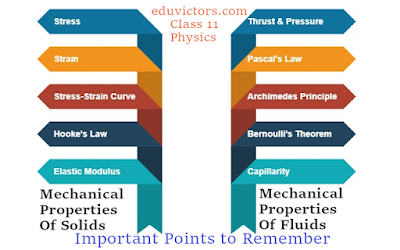# Class 11 - Physics - Mechanical Properties of Solids and Fluids - Important Points to Remember

1. Elasticity:

It is the property of a material by which it tries to regain its original configuration after the removal of the deforming force applied to it. Example of perfectly elastic solid, quartz, phosphor, bronze.

2. Plasticity:

It is the property of a body by virtue of which it does not regain its original shape and size even after the removal deforming force, is called plasticity.

3. Stress is the restoring force per unit area and strain is the fractional change in dimension. Stress can be:

- Normal or Longitudinal Stress,

- Tangential or Shearing Stress

- Hydraulic or Bulk Stress

4. When a deforming force acts on a body, the body undergoes a change in its shape and size. The ratio of a change in the configuration of the body to the original configuration is called strain.

Strain = $\frac{\text{Change in configuration}}{\text{Original Configuration}}$

5. When an object is under tension or compression, the Hooke's law takes the form F/A = Y ∆L/L where ∆L/L is the tensile or compressive strain of the object, and Y is the Young's modulus for the object. The stress is F/A.

6. Stress-strain curve

The stress-strain graph of a ductile metal is shown in figure. Initially, the stress strain graph is linear and it obeys the Hooke's Law upto the point called the proportional limit. At the yield point the material starts deforming under constant stress and it behaves like a viscous liquid.

7. Brittle, Ductile, Malleable solids:

There are some materials which break as soon as the stress is increased beyond the elastic limit. They are called brittle, e.g. glass, ceramics etc.

Materials which have large plastic range of extension are called ductile. Using this property materials can be drawn into thin wires, e.g. - Copper, aluminum etc.

Materials which can be hammered into thin sheets are called malleable eg. Gold, silver, lead, etc.

8. Work done in a stretched wire:

Elastic potential energy U stored in the wire.

$\therefore U = \frac{1}{2}F \times {\boldsymbol{l}} = \frac{1}{2}(stress) \times (strain) \times volume$

$\therefore U = \frac{1}{2}(\text{Young's modulus}) \times (strain)^{2}$

9. The Poisson effect is defined as a material's tendency to expand in a direction perpendicular to the compression direction. Poisson's ratio is a measure of the Poisson effect.

10. Poisson's Ratio is a dimensional less and unit less quantity.

Theoretical value of Poisson's Ratio :- -1 < σ < 1/2

Practical value of Poisson's Ratio :- 0 < σ < 1/2

11. The total normal force exerted by a liquid at rest on a surface in contact with it is called fluid thrust.

The molecules of a fluid kept in a container are in constant random motion and collide with each other and with walls of the container. Fluids in a container exert thrust in all directions.

12. The pressure of liquid at a point is the thrust (or normal force) exerted by the liquid at rest per unit area around that point. Pressure is a scalar quantity.

$Pressure=\frac{force}{area}$

13. The expression for pressure P = Pa + ρgh. Holds true if fluid is incompressible.

14. The gauge pressure is the difference of the actual pressure and the atmospheric pressure. P - Pa = Pg

15. Archimedes principle:

A body immersed in a fluid experiences an upward buoyant force equivalent to the weight of the fluid displaced by it.

16.Pascal's law:

It states that pressure in a fluid at rest is same at all points which are at the same height.

17.Viscosity:

The property of a fluid due to which it opposes the relative motion between its different layers is called viscosity.

18. Viscous force:

The force between the layers of a liquid opposing the relative motion is called viscous force.
$F= -\eta A\; \frac{\mathrm{d} v}{\mathrm{d} x}$; where η is a coefficient of viscosity and velocity gradient = $\frac{\mathrm{d} v}{\mathrm{d} x}$

19. Bernoulli's principle

states that as we move along a streamline, the sum of the pressure (P), the kinetic energy per unit volume (ρv2/2) and the potential energy per unit volume (ρgy) remains a constant.
P + ρv2/2 + ρgy = constant

20. Velocity of Efflux: v = √2gh

21. Surface tension

is a force per unit length (or surface energy per unit area) acting in the plane of interface between the liquid and the bounding surface.

22. Surface Energy:

The surface energy of a liquid can be defined as the excess potential energy per unit area of the liquid surface.

W = T∆A, where ∆A = increase in surface area,
T = Surface tension of the liquid

23. For a liquid drop (Only one free surface) :
For increasing radius 0 to R
Surface energy = T∆A = 4πT (R2 - 0)
= 4πR2T

24. For a soap bubble (For two free surfaces) :
For increasing radius R1 to R2 where R2 > R1
Surface energy = T∆A = T×2 (4πR22 - 4πR12)
= 8πT(R22 - R12)

Excess pressure in liquid drop: P = 2T/R
Excess pressure in the bubble formed in a liquid: P = 2T/R
Excess pressure in soap bubble: There is two free surfaces in a soap bubble so P = 4T/R

25. Angle of Contact:

At the point of contact of a liquid and a solid, if we draw a tangent to the surface of the liquid, it makes an angle with the side of the container, inside the liquid. This is called the angle of contact. Those liquids which wets solids and rises in capillary tubes have the angle of contact acute and the liquid surface is concave.   θ < 90⁰

26. Those liquids which neither rises nor falls in a capillary tube have angle of contact right angle their liquid surface is plane.

θ = 90°

27. Capillarity:

$T = \frac{r\left [ h+\left ( \frac{r}{3} \right ) \right ]dg}{2 \cos \theta}$

If the tube is very narrow, r/3 can be neglected in comparison with h, Hence $h = \frac{2T\cos\theta}{rdg}$ or $T = \frac{hrdg}{2\cos\theta}$